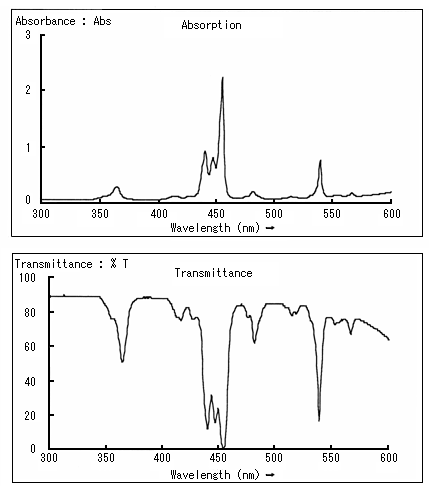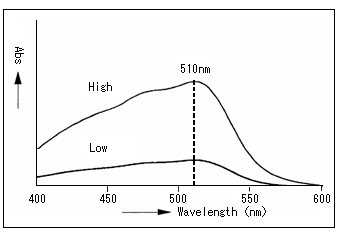# 5. Colorimetric Analysis (4)

## See a color (spectrum)

### What's a spectrum?

In a quantitative analysis, the amounts of light absorption of a substance were compared at a certain wavelength to determine concentration. Then, how much is the absorption at other wavelengths? "Spectrum" is a graph of the absorption of the substance at each wavelength.

Transmittance and absorption spectra can be measured with an ultraviolet-visible spectrophotometer.

In the spectrum, the horizontal axis indicates wavelength, and the vertical axis indicates transmittance and absorbance.

Look at the transmittance spectrum and the absorption spectrum indicated below to recall the relationship between transmittance and absorbance.### What can be determined from a spectrum?

Quantitative analysis of iron was performed in the preceding chapter. At which wavelength (nm) should measurement be conducted?

Higher absorption of the wavelength provides higher sensitivity; thus, it is desirable to select a wavelength with maximum absorption...

Here, the absorption spectrum comes in. See the spectrum below. This is an iron solution to which a coloring reagent was added. The absorption is highest at around 510 nm (the wavelength at which absorption reaches its peak is called absorption maximum wavelength). This tells us that the iron solution should be measured at around 510 nm.

The shape of this spectrum is unique to the iron solution to which a coloring reagent was added (the whole absorption intensity changes with concentration); thus, an unknown solution can be demonstrated to be an iron solution to which a coloring reagent was added if its spectrum measured corresponds to that of the following figure.

Thus, the properties of a substance can be investigated by measuring the spectrum.In addition, the chemical structure of a substance can also be known from absorption maximum wavelength, molar absorption coefficient, etc. of the ultraviolet-visible spectrum. (Qualitative analysis)